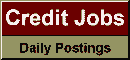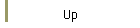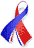DefaultRisk.com the web's biggest credit risk modeling resource.doi> search: A or B Export citation to:- HTML- Text (plain)- BibTeX- RIS- ReDIF

Measuring Marginal Risk Contributions in Credit Portfolios

by Paul Glasserman of Columbia Business School

Winter 2005/06

Abstract: We consider the problem of decomposing the credit risk in a portfolio into a sum of risk contributions associated with individual obligors or transactions. For some standard measures of risk - including value-at-risk and expected shortfall - the total risk can be usefully decomposed into a sum of marginal risk contributions from individual obligors. Each marginal risk contribution is the conditional expected loss from that obligor, conditional on a large loss for the full portfolio. We develop methods for calculating or approximating these conditional expectations. Ordinary Monte Carlo estimation is impractical for this problem because the conditional expectations defining the marginal risk contributions are conditioned on rare events. We develop three techniques to address this difficulty. First, we develop importance sampling estimators specifically designed for conditioning on large losses. Next, we use the analysis underlying the importance sampling technique to develop a hybrid method that combines an approximation with Monte Carlo. Finally, we take this approach a step further and develop a rough but fast approximation that dispenses entirely with Monte Carlo. We develop these methods in the Gaussian copula framework and illustrate their performance in multifactor models.

Published in: Journal of Computational Finance, Vol. 9, No. 2, (Winter 2005/06), pp. 1-41.

Books Referenced in this paper:  (what is this?)